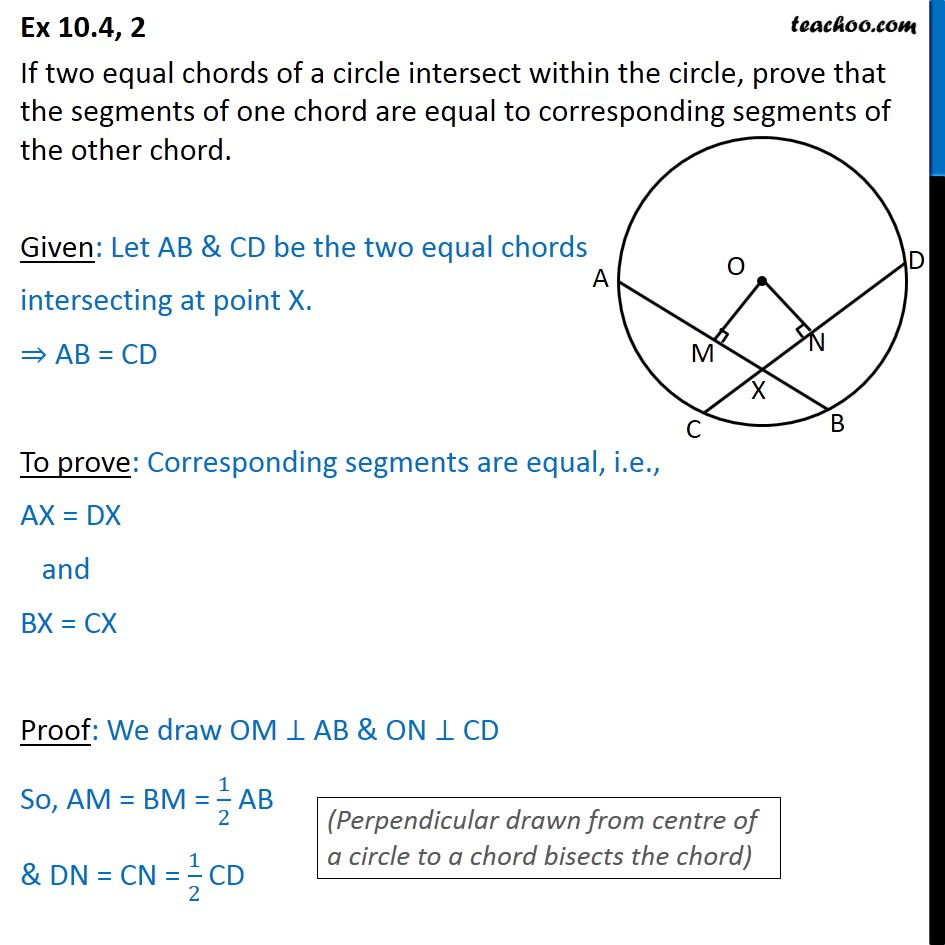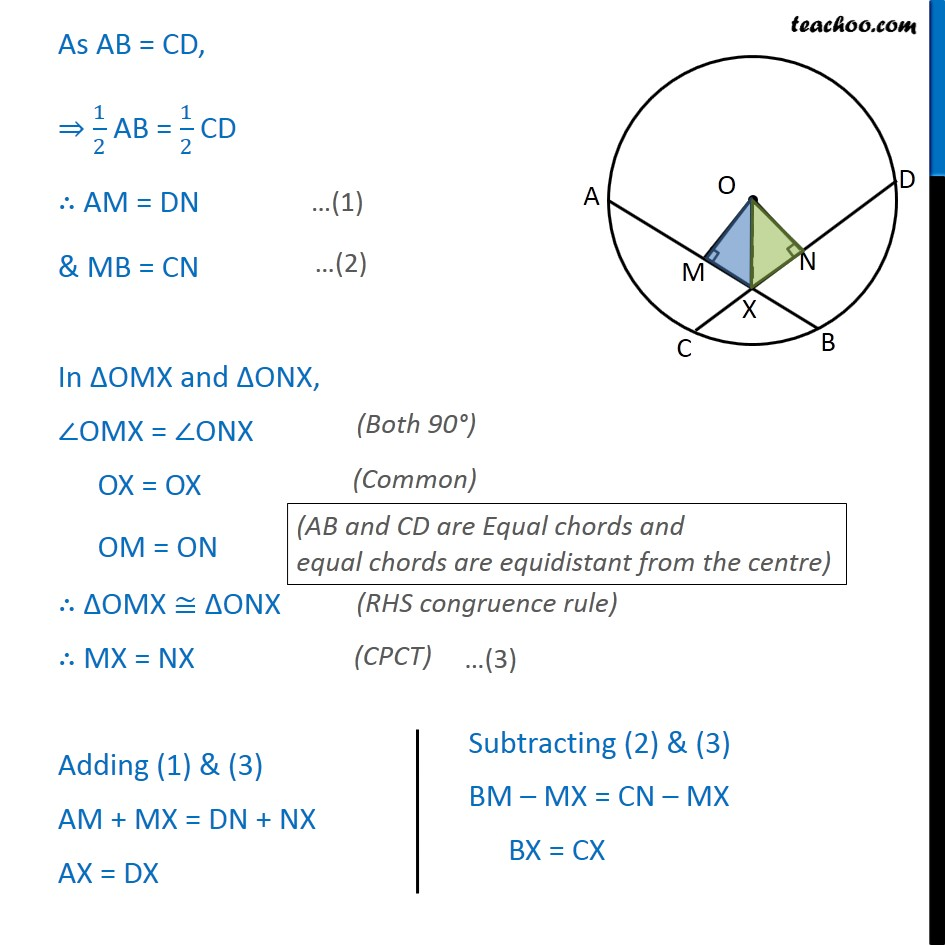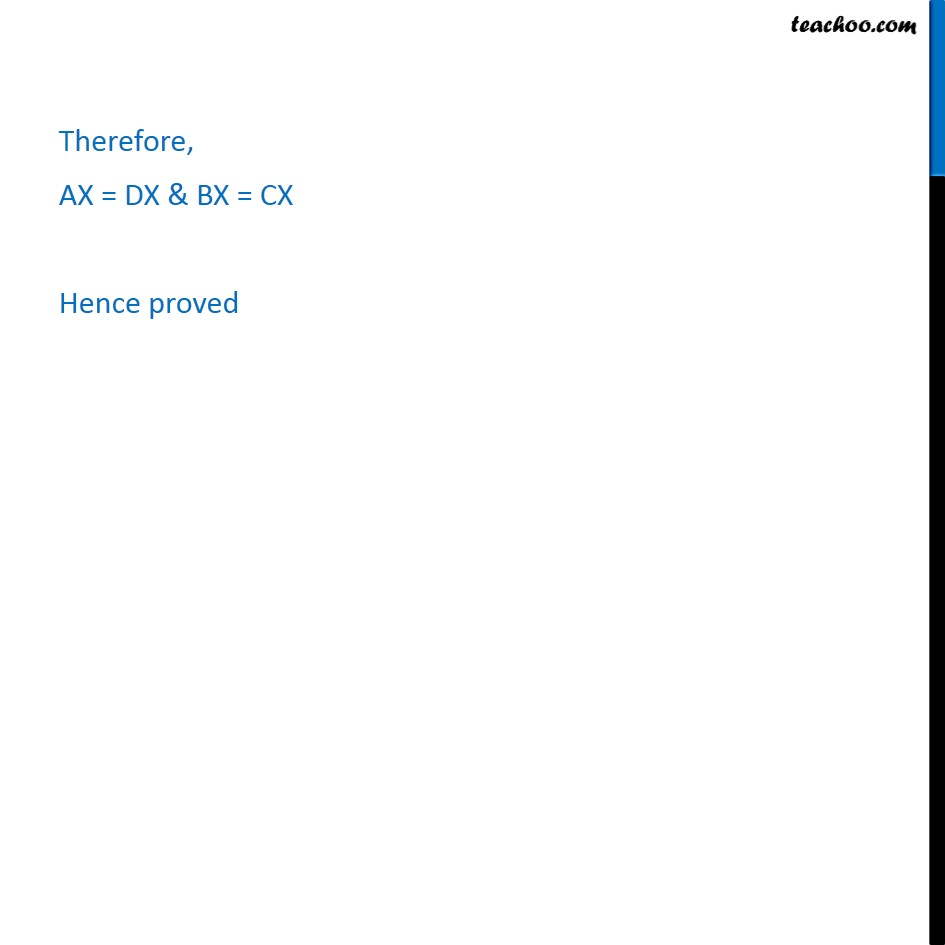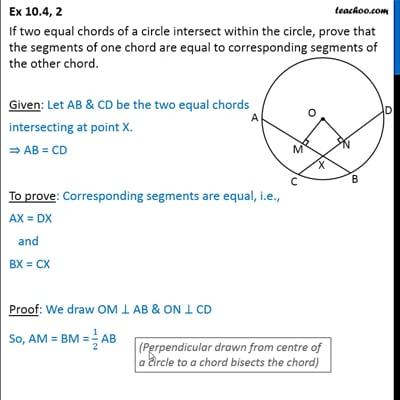Ex 10.4

Chapter 10 Class 9 Circles
Serial order wiseThis video is only available for Teachoo black users

Get live Maths 1-on-1 Classs - Class 6 to 12

### Transcript

Ex 10.4, 2 If two equal chords of a circle intersect within the circle, prove that the segments of one chord are equal to corresponding segments of the other chord. Given: Let AB & CD be the two equal chords intersecting at point X. ⇒ AB = CD To prove: Corresponding segments are equal, i.e., AX = DX and BX = CX Proof: We draw OM ⊥ AB & ON ⊥ CD So, AM = BM = 1/2 AB & DN = CN = 1/2 CD As AB = CD, ⇒ 1/2 AB = 1/2 CD ∴ AM = DN & MB = CN In ΔOMX and ΔONX, ∠OMX = ∠ONX OX = OX OM = ON ∴ ΔOMX ≅ ΔONX ∴ MX = NX Adding (1) & (3) AM + MX = DN + NX AX = DX Therefore, AX = DX & BX = CX Hence proved